# Plot classification probability¶

Plot the classification probability for different classifiers. We use a 3 class dataset, and we classify it with a Support Vector classifier, L1 and L2 penalized logistic regression with either a One-Vs-Rest or multinomial setting, and Gaussian process classification.

Linear SVC is not a probabilistic classifier by default but it has a built-in calibration option enabled in this example (probability=True).

The logistic regression with One-Vs-Rest is not a multiclass classifier out of the box. As a result it has more trouble in separating class 2 and 3 than the other estimators.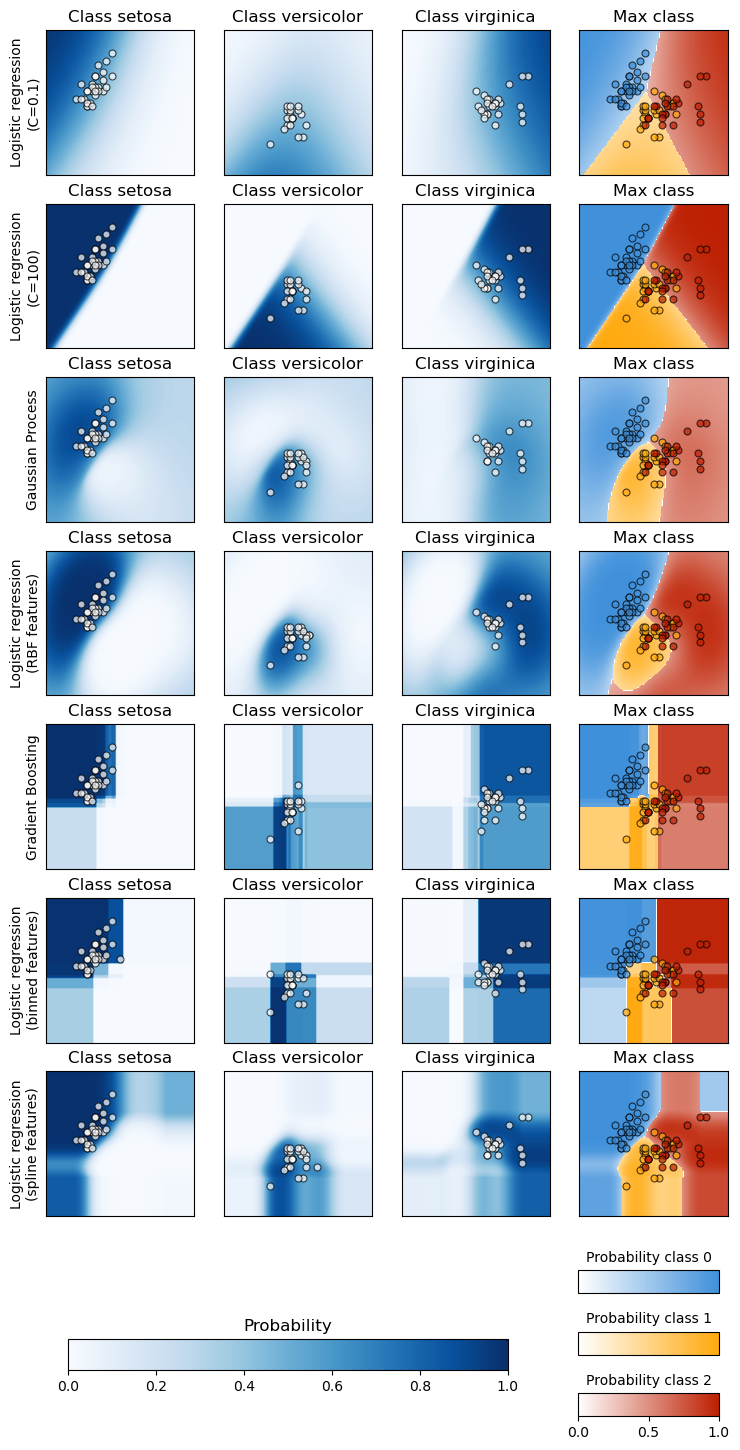Accuracy (train) for L1 logistic: 83.3%
Accuracy (train) for L2 logistic (Multinomial): 82.7%
Accuracy (train) for L2 logistic (OvR): 79.3%
Accuracy (train) for Linear SVC: 82.0%
Accuracy (train) for GPC: 82.7%


# Author: Alexandre Gramfort <alexandre.gramfort@inria.fr>

import matplotlib.pyplot as plt
import numpy as np
from matplotlib import cm

from sklearn import datasets
from sklearn.gaussian_process import GaussianProcessClassifier
from sklearn.gaussian_process.kernels import RBF
from sklearn.inspection import DecisionBoundaryDisplay
from sklearn.linear_model import LogisticRegression
from sklearn.metrics import accuracy_score
from sklearn.svm import SVC

X = iris.data[:, 0:2]  # we only take the first two features for visualization
y = iris.target

n_features = X.shape

C = 10
kernel = 1.0 * RBF([1.0, 1.0])  # for GPC

# Create different classifiers.
classifiers = {
"L1 logistic": LogisticRegression(
C=C, penalty="l1", solver="saga", multi_class="multinomial", max_iter=10000
),
"L2 logistic (Multinomial)": LogisticRegression(
C=C, penalty="l2", solver="saga", multi_class="multinomial", max_iter=10000
),
"L2 logistic (OvR)": LogisticRegression(
C=C, penalty="l2", solver="saga", multi_class="ovr", max_iter=10000
),
"Linear SVC": SVC(kernel="linear", C=C, probability=True, random_state=0),
"GPC": GaussianProcessClassifier(kernel),
}

n_classifiers = len(classifiers)

fig, axes = plt.subplots(
nrows=n_classifiers,
ncols=len(iris.target_names),
figsize=(3 * 2, n_classifiers * 2),
)
for classifier_idx, (name, classifier) in enumerate(classifiers.items()):
y_pred = classifier.fit(X, y).predict(X)
accuracy = accuracy_score(y, y_pred)
print(f"Accuracy (train) for {name}: {accuracy:0.1%}")
for label in np.unique(y):
# plot the probability estimate provided by the classifier
disp = DecisionBoundaryDisplay.from_estimator(
classifier,
X,
response_method="predict_proba",
class_of_interest=label,
ax=axes[classifier_idx, label],
vmin=0,
vmax=1,
)
axes[classifier_idx, label].set_title(f"Class {label}")
# plot data predicted to belong to given class
axes[classifier_idx, label].scatter(
)
axes[classifier_idx, label].set(xticks=(), yticks=())
axes[classifier_idx, 0].set_ylabel(name)

ax = plt.axes([0.15, 0.04, 0.7, 0.02])
plt.title("Probability")
_ = plt.colorbar(
cm.ScalarMappable(norm=None, cmap="viridis"), cax=ax, orientation="horizontal"
)

plt.show()


Total running time of the script: (0 minutes 1.332 seconds)

Related examples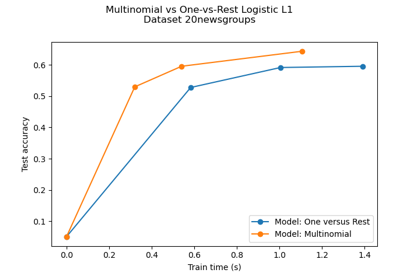Multiclass sparse logistic regression on 20newgroups

Multiclass sparse logistic regression on 20newgroups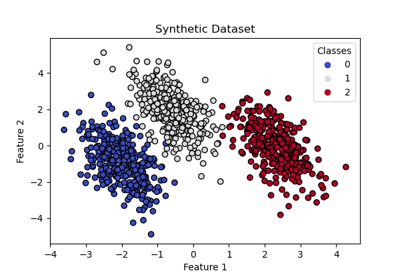Plot multinomial and One-vs-Rest Logistic Regression

Plot multinomial and One-vs-Rest Logistic Regression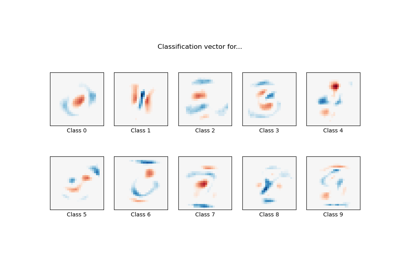MNIST classification using multinomial logistic + L1

MNIST classification using multinomial logistic + L1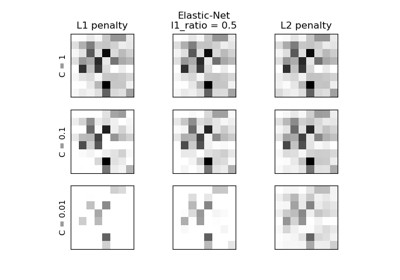L1 Penalty and Sparsity in Logistic Regression

L1 Penalty and Sparsity in Logistic RegressionLogistic Regression 3-class Classifier

Logistic Regression 3-class Classifier

Gallery generated by Sphinx-Gallery